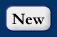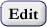## ValueTypeCV Values

Used to populate the ValueType field in the Variables tableTerm DefinitionCalibration Value A value used as part of the calibration of an instrument at a particular time.Derived Value Data are the result of a mathematical transformation where there is some subjectivity in the selected equation form and/or where parameters are typically fitted. A rating curve relating stage to discharge is a transformation that fits this definition; therefore stage is observed but discharge is derived.Estimated Value Values that are roughly calculated, judged, or approximated. Values may result from professional judgement or from ancillary data.Field Observation Data reflect the measurement the sensor is designed to make, reported at the frequency of data collectionModel Simulation Result Values generated by a simulation modelSample Observation that is the result of analyzing a sample in a laboratoryUnknown The value type is unknown